top of page# In the matrix form above system of equation is written as:

Linear System of Equations# Vector X is such that on linear transformation with matrix M, it lands on vector D.# Here X is unknown while D and M are known. So if we perform inverse transformation of M on D, we can find X.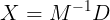• # If vector D lies on the same line as iT and jT, then system of equation has infinitely many solution.

Consistency of system• # If vector D does not lie on the same line as iT and jT, then system of equation is inconsistent amd no solution exists.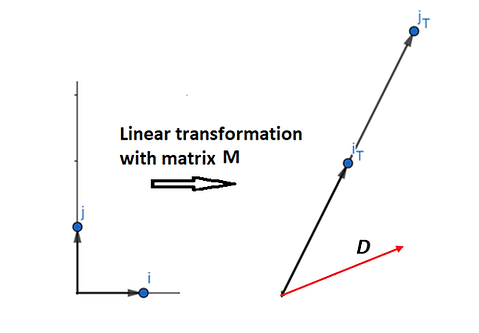• # iT and jT (unit vector i and j after transformation) remain in one plane but are not collinear. Number of dimension in the output of transformation is 2, hence rank is 2.

Rank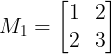• # iT and jT become collinear after transformation. No of dimension in the output is reduced to 1, hence rank is 1.• # iT and jT are reduced to a point after linear transformation. Since origin remain fixed in linear transformation, hence iT and jT both shift to origin. For this case rank of the matrix is zero.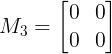# Consider a linear system of equation MX = D. For this system augmented matrix MA is defined as# D) 4

Previous years questions# Solution: Consider a 3x4 real matrix A as:# Solution: Consider matrix A and B as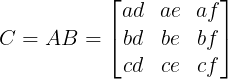# D) √2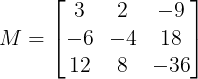# where;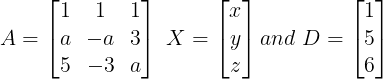# For a = 4, augmented matrix A:D is# Convert to echelon form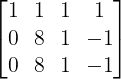# Similarly for a = -3, augmented matrix A:D in echelon form is# GATE 2016: The solution to the system of equations as given below is# Solution: For system of equation Ax = B, x is found out by# Matrix of cofactors of A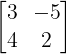# Inverse of A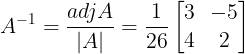# where;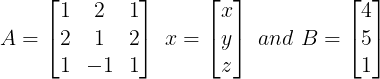# Augmented matrix A:B is# Echelon form of A:B is# Solution: System of equation in matrix form is written as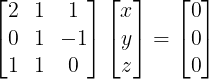# Solution: System of equation in matrix form Ax = B is written as: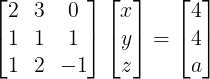# Augmented matrix A:B is written as# Augmented matrix in echelon form is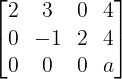bottom of page